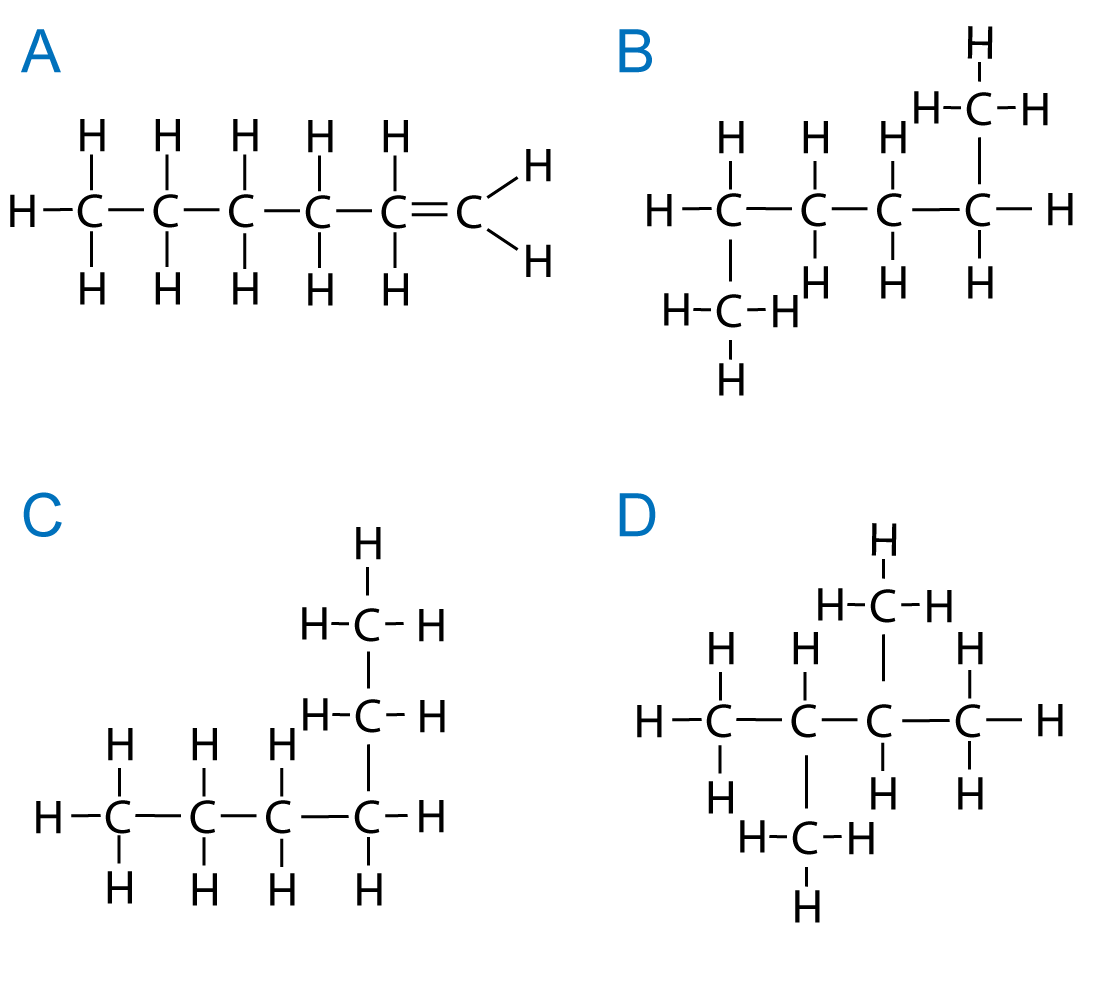10 minutes maximum! Can you do it in 5? Q1-3:Under certain conditions ethane will react slowly with bromine. 1. The conditions required for this reaction are ... A.   a temperature of 300oC B.   a catalyst of alumina or silica C.   sunlight or u.v. light D.   a pressure of 60atm ABCD 2. The correct equation for the reaction between ethane and bromine is ... A.  C2H6 + Br2  →  C2H5Br + HBr B.  C2H6 + Br2  →  C2H4Br2 + H2 C.  C2H6 + Br2  →  C2H6Br2  D.  C2H4 + Br2  →  C2H4Br2 ABCD 3. The reaction between ethane and bromine is a(n) ? addition substitution displacement cracking reaction. 4. Isomers are molecules with the ... A.   same general formula but a different structural formula B.   same molecular formula but a different structural formula C.   same general formula but a different molecular formula D.   same molecular formula but a different functional group ABCD 5. Hexane has the structure:Which one of the following is an isomer of hexane?ABCD 6. Which of the following structures, X, Y and Z are isomers of C4H8 ?A.   X only B.   X and Y only C.   X and Z only D.   X, Y and Z ABCD Q7-10 refer to the following compounds, P, Q, R and S:7. Which compounds are isomers? A.   P and Q B.   P and R C.   R and S D.   Q and S ABCD 8. Which compound(s) will decolourise bromine water immediately? A.   P and Q B.   P and R C.   R and S D.   Q and S ABCD 9. Which compound(s) can form addition polymers? A.   P and Q B.   P and R C.   R and S D.   Q and S ABCD 10. Which compounds are different members of the same homologous series? A.   P and Q B.   P and R C.   R and S D.   Q and S ABCD